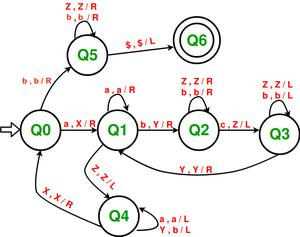# Construct a Turing machine for L = {aibjck | i*j = k; i, j, k ≥ 1}

• Difficulty Level : Expert
• Last Updated : 18 Oct, 2021

Prerequisite – Turing Machine
In a given language, L = {aibjck | i*j = k; i, j, k ≥ 1}, where every string of ‘a’, ‘b’ and ‘c’ has a certain number of a’s, then a certain number of b’s and then a certain number of c’s. The condition is that each of these 3 symbols should occur at least once. ‘a’ and ‘b’ can occur however many times, but the occurrences of ‘c’ must be equal to the product of occurrences of ‘a’ and occurrences of ‘b’. Assume that string is ending with ‘\$’.

Examples –

```Input: a a b b b c c c c c c
Here a = 2, b = 3, c = 2 * 3 = 6
Output: ACCEPTED

Input: a a b b c c c
Here a = 2, b = 2, c = 3 but c should be 4
Output: NOT ACCEPTED ```

Approach used – Scan the input from the left.

1. First, replace an ‘a’ with ‘X’ and move 1 step right. Then skip all the a’s and move right.
2. When the pointer reaches the first ‘b’ stop. Replace one ‘b’ with ‘Y’, then move right skipping all intermediate b’s and corresponding to the replaced ‘b’ now replace one ‘c’ with ‘Z’ and move left.
3. Now move towards the left skipping all ‘Z’ and ‘b’ in the way.
4. When the pointer reaches the most recent ‘Y’ move right.
5. If the pointer is pointing at ‘b’ then repeat steps 2 to 4, else if the pointer is pointing at ‘Z’ then move towards left while replacing all ‘Y’ to ‘b’ and skipping all a’s.
6. When the pointer comes to the most recent ‘X’ move one step right.
7. If the pointer is still pointing to ‘a’ then repeat all the above steps, else if the pointer is at ‘b’ then move towards right skipping all ‘b’ and ‘Z’.
8. When \$ is reached then move left. The string is ACCEPTED.My Personal Notes arrow_drop_up